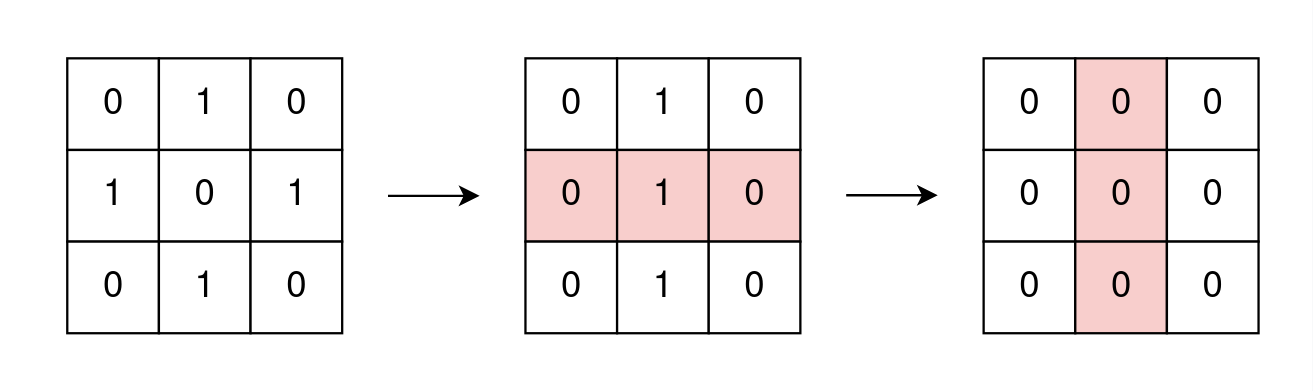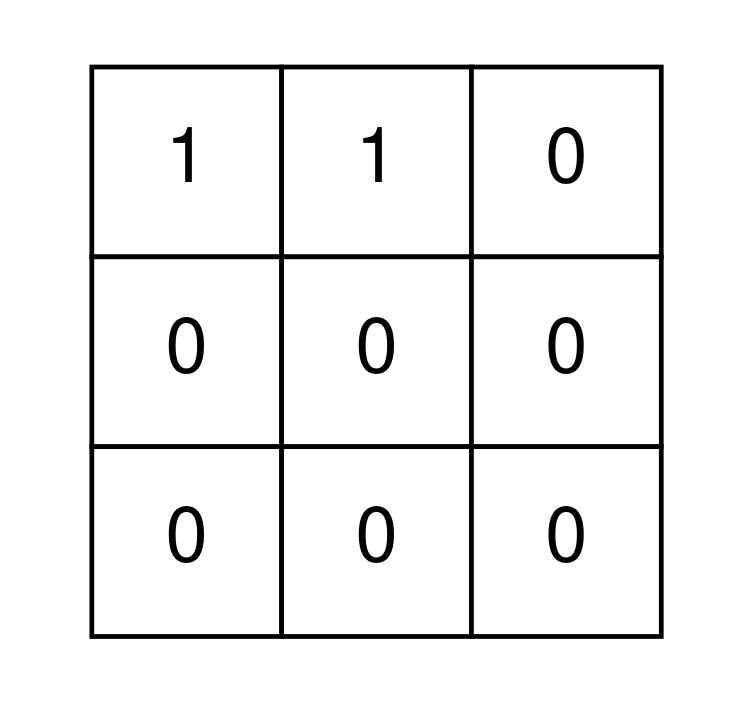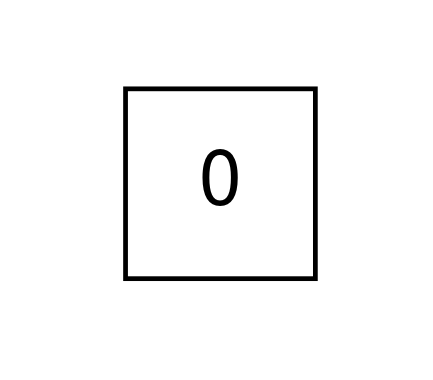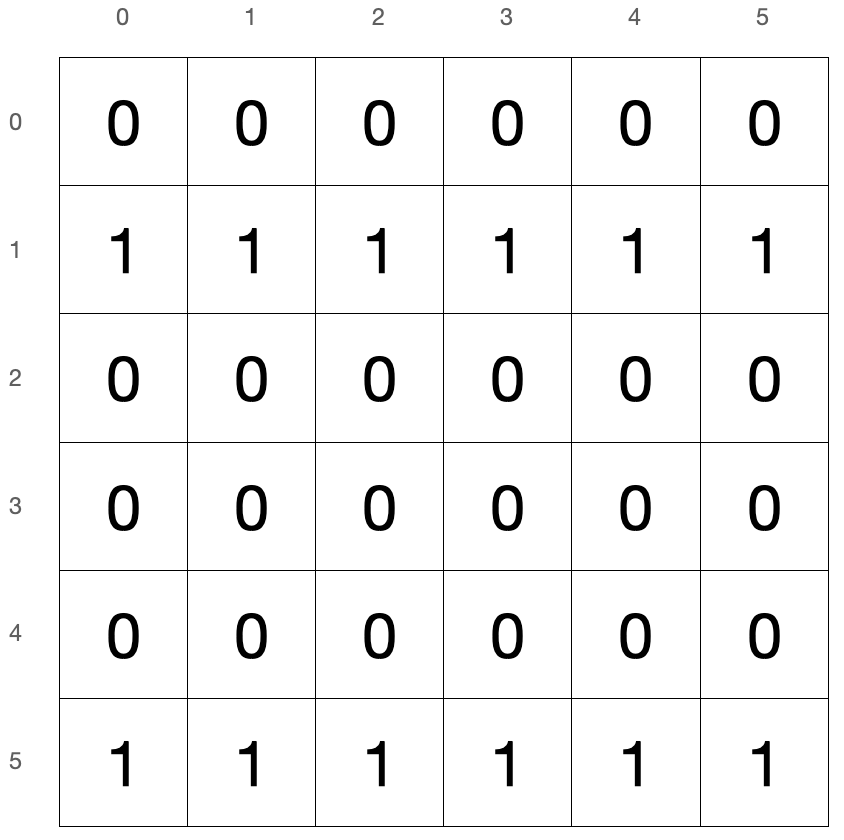# LeetCode 2128. Remove All Ones With Row and Column Flips Solution

You are given an m x n binary matrix grid.

In one operation, you can choose any row or column and flip each value in that row or colum (i.e., changing all $0$'s to $1$'s, and all $1$'s to $0$'s).

Return true if it is possible to remove all 1's from grid using any number of operations or false otherwise.

Example 1:Input: grid = [[0,1,0],[1,0,1],[0,1,0]]
Output: true
Explanation: One possible way to remove all $1$'s from grid is to:

• Flip the middle row
• Flip the middle column

Example 2:Input: grid = [[1,1,0],[0,0,0],[0,0,0]]
Output: false
Explanation: It is impossible to remove all $1$'s from grid.Input: grid = []
Output: true
Explanation: There are no $1$'s in grid.

Constraints:

• m == grid.length
• n == grid[i].length
• 1 <= m, n <= 300
• grid[i][j] is either $0$ or $1$.

## Brute Force

The first observation we can make is that for every row/column, we will either flip that row/column once or zero times. This is because if we flip a row/column twice, it will have no effect on the grid.

With this in mind, for each row and column, we can either choose to flip it or not flip it. If we brute force every combination, we will take $O(MN)$ to simulate each combination. Since there are $O(2^{M+N})$ combinations, this gives us a time complexity of $O(2^{M+N}\times MN)$. Let's look for a faster solution as this is way too slow.

## Full Solution

Let's call grid reachable if it's possible to apply some row/column flips to remove all $0$'s.

We can observe that if each row/column is flipped at most once, there is only one set of rows and columns that can be flipped to set all cells to $0$ (assuming a solution exists). Let's call these necessary rows and columns.

To check whether or not grid is reachable, we'll first flip all necessary columns, and then the necessary rows.

Let's first assume that we already flipped all necessary columns on grid. We can observe that grid is now reachable by only row flips if all rows have the same integer (i.e. all $0$'s or all $1$'s).

### ExampleHere, we have a grid reachable by only row flips as all rows have the same integer. We can remove all $1$'s from grid by flipping rows with all $1$'s. Here, we will flip rows $1$ and $5$.

Our goal is to figure out a way to flip some set of columns such that grid will only contain rows with all $0$'s or all $1$'s.

With that in mind, our algorithm will go as follows. First, find all cells in the first row of grid with a $1$. For every cell (0,j) in the first row that's a $1$, let's flip the $j^{th}$ column so that the cell (0,j) becomes a $0$. This is important as it causes the first row to have all $0$'s. At this point there are two cases to consider.

• The first case is that the rest of the rows have all $0$'s or all $1$'s. In this case, we can conclude that grid is reachable and we'll return true.

• The second case is that there exists a row that has a mix of $0$'s and $1$'s, which we don't want. In this case, we'll return false since we can observe that it's impossible to get all rows to have all $0$'s or all $1$'s. This is because if we try to change the other rows to have all $0$'s or $1$'s with more column flips, the content of the first row will change and it will no longer have all $0$'s.

### Time Complexity

In the worst scenario, we will perform $O(M)$ row flips and $O(N)$ column flips. Since row flips take $O(N)$ to simulate and column flips take $O(M)$ to simulate, our total time complexity is $O(NM)$.

Time Complexity: $O(NM)$.

### Space Complexity

Since we don't actually use additional arrays or lists, our space complexity is $O(1)$.

Space Complexity: $O(1)$.

## C++ Solution

1class Solution {
2   public:
3    bool removeOnes(vector<vector<int>>& grid) {
4        int m = grid.size();  // get dimensions of grid
5        int n = grid.size();
6        for (int i = 0; i < n; i++) {  // flip columns so that first row only has 0's
7            if (grid[i] == 1) {
8                for (int j = 0; j < m; j++) {  // flips a column
9                    grid[j][i] = 1 - grid[j][i];
10                }
11            }
12        }
13        bool ans = true;
14        for (int i = 0; i < m; i++) {  // checks if each row has all 0's or all 1's
15            int sum = 0;
16            for (int j = 0; j < n; j++) {
17                sum += grid[i][j];
18            }
19            if (sum == 0 || sum == n) {
20                continue;
21            }
22            ans = false;
23        }
24        return ans;
25    }
26};

## Java Solution

1class Solution {
2    public boolean removeOnes(int[][] grid) {
3        int m = grid.length; // get dimensions of grid
4        int n = grid.length;
5        for (int i = 0; i < n; i++) { // flip columns so that first row only has 0's
6            if (grid[i] == 1) {
7                for (int j = 0; j < m; j++) { // flips a column
8                    grid[j][i] = 1 - grid[j][i];
9                }
10            }
11        }
12        boolean ans = true;
13        for (int i = 0; i < m; i++) { // checks if each row has all 0's or all 1's
14            int sum = 0;
15            for (int j = 0; j < n; j++) {
16                sum += grid[i][j];
17            }
18            if (sum == 0 || sum == n) {
19                continue;
20            }
21            ans = false;
22        }
23        return ans;
24    }
25}

## Python Solution

1class Solution:
2    def removeOnes(self, grid: List[List[int]]) -> bool:
3        m = len(grid)  # get dimensions of grid
4        n = len(grid)
5        for i in range(n):  # flip columns so that first row only has 0's
6            if grid[i] == 1:
7                for j in range(m):  # flips a column
8                    grid[j][i] = 1 - grid[j][i]
9        ans = True
10        for i in range(m):  # checks if each row has all 0's or all 1's
11            sum = 0
12            for j in range(n):
13                sum += grid[i][j]
14            if sum == 0 or sum == n:
15                continue
16            ans = False
17        return ans
18